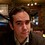# Help animating moving a line with mouse

Hi,
Can anyone please help with this function? I am trying to move the line with mouse when it is pressed, by updating the y-coordinates of the line. It works ok on a small figure window, but there is great lag when using full screen. I want to use animation or blitting functions to make the update faster.
The code is attached here.

``````import numpy as np
import matplotlib.pyplot as plt
import math
from scipy.interpolate import interp1d

class MoveLine:
def __init__(self, line):
self.line = line
self.pressed = False
self.xs = list(line.get_xdata())
self.ys = list(line.get_ydata())
self.cidpress = line.figure.canvas.mpl_connect('button_press_event', self.on_press)
self.cidmotion = line.figure.canvas.mpl_connect('motion_notify_event', self.on_motion)
self.cidrelease = line.figure.canvas.mpl_connect('button_release_event', self.on_release)

"""callback for button press and motion together"""
def on_press(self, event):
if event.inaxes!=self.line.axes: return
self.pressed = True
self.line.figure.canvas.draw()

def on_motion(self, event):
if event.inaxes!=self.line.axes: return
if self.pressed:
xs = event.xdata
# xs = math.ceil(xs)
ys = event.ydata
PointDiff = abs(InterpSegX-xs)
PointChanged = np.where(PointDiff <=3)
PointChanged = np.array(PointChanged)
# PointChanged = math.ceil(PointChanged)
InterpSegY[PointChanged] = ys
self.line.set_ydata(InterpSegY)
self.line.figure.canvas.draw()

def on_release(self, event):
self.pressed = False
self.line.figure.canvas.draw()

def disconnect(self):
"""disconnect all callbacks"""
self.line.figure.canvas.mpl_disconnect(self.cidpress)
self.line.figure.canvas.mpl_disconnect(self.cidrelease)

fig, ax = plt.subplots()
linex = list(range(0,1536))#creating line to plot with x and y data
linex = np.array(linex)

rows, cols = (1536, 1)
liney = [*cols]*rows
liney=np.array(liney)
liney=liney[:,0]
"""creatint interpolation of line"""
segx=np.linspace(1,1535, 200).astype(int)
InterpSegX = np.interp(segx,linex,linex)

InterpSegx = np.array(InterpSegX).astype(int)
InterpSegY = np.interp(segx,linex,liney)

InterpSegY = np.array(InterpSegY).astype(int)

line, = ax.plot(InterpSegX, InterpSegY, linewidth=0.5)
moveline = MoveLine(line)
plt.show()
``````

Thanks for the link! I had looked into it, the issue I am getting is that the line would not update unless I stopped dragging the mouse, but I wanted it to update in real time and not wait until the on_release to happen.

You will want to put the blitting logic inside of the `on_motion` callback. What backend are you using?

A number of our builtin widgets have the functionality, so those may be good places to look (`matplotlib/widgets.py`) for reference.

Thanks for the suggestion. I use the backend qt4agg.
I will check out the widgets that you have suggested.

···

On Tue, Aug 31, 2021 at 2:02 PM tacaswell via Matplotlib <nobody@discourse.matplotlib.org> wrote:tacaswell
August 31

You will want to put the blitting logic inside of the `on_motion` callback. What backend are you using?

A number of our builtin widgets have the functionality, so those may be good places to look (`matplotlib/widgets.py`) for reference.

Visit Topic or reply to this email to respond.